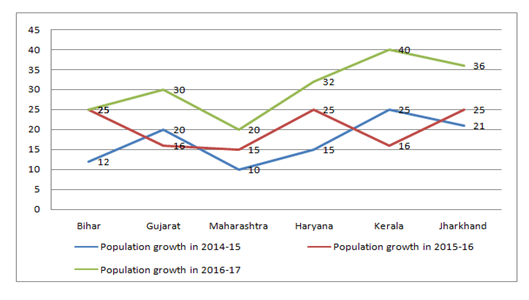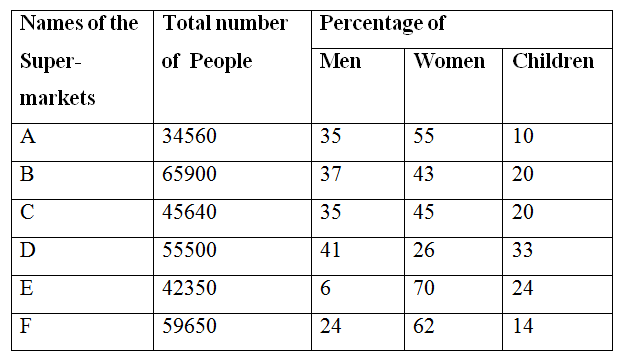# Quantitative Aptitude Questions (Data Interpretation) for IBPS RRB Assistant 2018 Day-170

Dear Readers, IBPS RRB is conducting Online Examination for the recruitment of clerical cadre To enrich your preparation here we have providing new series of Data Interpretation – Quantitative Aptitude Questions. Candidates those who are appearing in IBPS RRB Assistant Exams can practice these Quantitative Aptitude average questions daily and make your preparation effective.

[WpProQuiz 3272]

Click “Start Quiz” to attend these Questions and view Solutions

Directions (Q. 1 – 5): Study the following information carefully and answer the given questions.

Following line-graph shows the percentage growth of population of six states in three years.1) If the population of Haryana in the year 2017 is 2087250, then find its population in the year 2014?

a) 11 lakh

b) 10.5 lakh

c) 13.5 lakh

d) 12.75 lakh

e) 14.25 lakh

2) If the population of Maharashtra and that of Haryana were equal in the year 2014, then find the ratio of the population of Maharashtra to that of Haryana in 2016?

a) 25 : 22

b) 22 : 31

c) 23 : 32

d) 21 : 29

e) 22 : 25

3) If in the year 2017, the populations of Bihar and Gujarat are 1388800 and 1302912 respectively, the population of Gujarat in the year 2014 is approximately what percentage of the population of Bihar in the year 2014?

a) 70%

b) 80%

c) 95%

d) 90%

e) 100%

4) If the population of Maharashtra in the year 2014 was 8.5 lakh, then find the population of Maharashtra in the year 2017?

a) 10.786 lakh

b) 13.634 lakh

c) 12.903 lakh

d) 15.406 lakh

e) 12.107 lakh

5)  If the population of Kerala and Jharkhand in the year 2014 was 12.5 lakh and 10 lakh respectively, then find the difference between the population of Kerala and the population of Jharkhand in the year 2017?

a) 4.915 lakh

b) 4.805 lakh

c) 4.385 lakh

d) 4.275 lakh

e) None of these

Directions (Q. 6 – 10): Study the following table carefully and answer the questions given follow:

Number of people visiting six different Super-markets and the percentage of Men, Women and Children visiting those Super-markets6) The Number of men visiting Super-market D is approximately what percent of the total number of people visiting all the Super-markets together?

a) 26 %

b) 22 %

c) 17 %

d) 8 %

e) 33 %

7) What is the total number of children visiting Super-markets B, D and F together?

a) 38515

b) 28479

c) 39846

d) 22308

e) None of these

8) What is the average number of women visiting all the Super-markets except from Super markets C and F?

a) 22855

b) 25720

c) 20850

d) 21255

e) None of these

9) The Number of children visiting Super-market C is what percentage more/less than the number of children visiting Super- market F?

a) 9 % more

b) 15 % more

c) 9 % less

d) 15 % less

e) 30 % more

10) What is the ratio of number of women visiting Super-market A to that of Super-market C?

a) 35 : 37

b) 245 : 316

c) 352 : 377

d) 1056 : 1141

e) None of these

Explanation:

Population in 2014

= > 2087250 × (100/115) × (100/125) × (100/132)

= > 11 lakh

Explanation:

Let the population of Maharashtra and Haryana be x at the beginning of 2014.

Population in Maharashtra 2016 = x × 110/100 × 115/100

Population in Haryana 2016 = x × 115/100 × 125/100

Required ratio = 110 : 125 = 22 : 25

Explanation:

Population in Bihar 2014

= 1388800 × 100/112 × 100/125 × 100/125

= 793600 lakh

Population in Gujarat 2014

= 1302912 × 100/120 × 100/116 × 100/130 = 7.2 lakh

Required percentage = (720000/793600) × 100 = 90%

Explanation:

Population in 2017

= 850000 × 110/100 × 115/100 × 120/100

= 1290300

= 12.903 lakhs

Explanation:

Kerala in 2017

= 12.5 × 125/100 × 116/100 × 140/100

= 25.375 lakh

Jharkhand in 2017

= 10 × 121/100 × 125/100 × 136/100

= 20.57 lakh

Required difference = 25.375 – 20.57 = 4.805 lakh

Explanation:

Number of men visiting supermarket D

= > 41% of 55500 = 41 × 555 = 22755

Total number of people visiting all the supermarkets together

= > 34560 + 65900 + 45640 + 55500 + 42350 + 59650 = 303600

Required % = (22550/303600) × 100 = 7.49 % = 8 %

Explanation:

Total number of children visiting supermarket B and D together

= > (20/100)*65900 + (33/100)*55500 + (14/100)*59650

= > 13180 + 18315 + 8351 = 39846

Explanation:

Total number of women

= > (55/100)*34560 + (43/100)*65900 + (26/100)*55500 + (70/100)*42350

= > 19008 + 28337 + 14430 + 29645 = 91420

Required average = 91420/4 = 22855

Explanation:

Number of children visiting supermarkets C

= > 20% of 45640 = 20*(45640/100) = 9128

Number of children visiting supermarket F

= > 14% of 59650 = (14/100)*59650 = 8351

Therefore required percentage = [(9128 – 8351)/8351] × 100

= > (777/8351)*100

= > 9 % more

Explanation:

The number of women visiting Super-market A

= > 34560*(55/100) = 19008

The number of women visiting Super-market C

= > 45640*(45/100) = 20538

Required ratio = 19008 : 20538 = 1056 : 1141

Daily Practice Test Schedule | Good Luck

 Topic Daily Publishing Time Daily News Papers & Editorials 8.00 AM Current Affairs Quiz 9.00 AM Quantitative Aptitude “20-20” 11.00 AM Vocabulary (Based on The Hindu) 12.00 PM General Awareness “20-20” 1.00 PM English Language “20-20” 2.00 PM Reasoning Puzzles & Seating 4.00 PM Daily Current Affairs Updates 5.00 PM Data Interpretation / Application Sums (Topic Wise) 6.00 PM Reasoning Ability “20-20” 7.00 PM English Language (New Pattern Questions) 8.00 PM